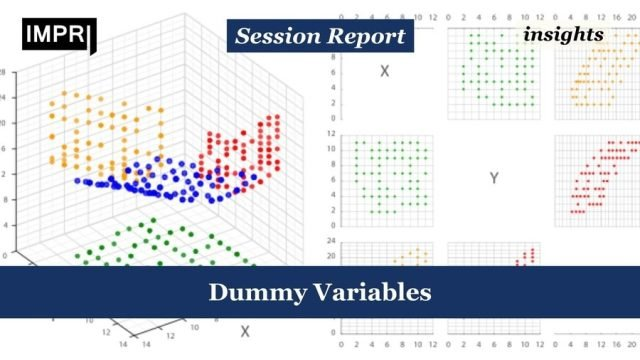Home Insights Dummy Variables – IMPRI Impact And Policy Research Institute

# Dummy Variables – IMPRI Impact And Policy Research Institute

10
0## Session ReportLiya Jomon

On the 4th day of the educational session initiated by IMPRI, Professor Nilanjan Banik, a professor and program director at Mahendra University, Hyderabad. He is also a visiting professor at IMPRI. Professor Nilanjan enlightened us with his knowledge on the topic of Dummy Variables.

The session started by giving a good brief about the following topics that were previously taken upon in a session.

The topics were:

Explain the Anova, Explain Mean squares, ESS, RSS, TSS, Derivation of F both for model components and Y bar-vis-a-vis Y-hat, How to calculate t-statistics, check for endogeneity, corollary, the covariance between Y-hat with error, Prediction/forecast, interval estimation of parameters and Ys, Two types of prediction; prediction for the mean value of Y and prediction for individual Y, Prediction error higher for the individual Ys than for the mean Y, Prediction Y using dummy variables.

After a good brief, Prof. Nilanjan started by specifying the three main reasons why dummy variable is used.

The reasons were:

1. Change in slope and intercept.
2. Interpretation of multiple categories of dummy variables.
3. Deseasonalize the data. Y-bas plus error.

Prof. Nilanjan explained each point in an efficient manner, the first point being:

### Change in Slope and Intercept due to Dummy Variables:

#### Change in intercept-

Two graphs were used in this explanation with represented coincident regressions and parallel regressions. Using these two visual interpretations, it was highlighted how in parallel regressions, due to an exogenous shock there has been an increase in income, and thus a subsequent increase in savings also, this makes the intercept go higher in the graph.

As savings is a function of income, thus with a jump in the income, there will be a jump in savings also. This jump in the two variables, makes the intercept increase.

#### Change in slope-

To explain the change in slope, two graphs were used which highlighted concurrent regressions and dissimilar regressions.

In the first graph, it was observed how the slope increased.

A formula was used which was pinned down by pooling all the observations and running just one multiple regression. The formula was

Yt =α1+α2Dt+β1Xt+β2(DtXt)+ut

where

Y = savings

X = income

t = time

D = 1 for observations in 1982-1995

= 0, otherwise (i.e., for observations in 1970-1981)

The interactive dummy was mentioned. Later in the session, an image was shared to explain the concept of multiple dummies, where all the variables were well explained.

Later in the session, Prof. Nilanjan introduced the topic of Heteroscedasticity, which focused on:

1. Problem with Heteroscedasticity.
2. How to detect Heteroscedasticity
3. Goldfeld-Quandt test, Breusch-Pagan test and White’s test in excel framework.
4. How to perform these tests in Excel format.

An Excel sheet was shared to explain the topic and demonstrated how to use dummy variables. Various operations were done in the Excel sheet and the corresponding responses were explained by Prof. Nilanjan who highlighted how each topic is efficient.

The operations were done using four dummies, each response was focused upon and this complimented the process of working with multiple dummy variables.

Using the Excel data, the previously discussed concept of change slope and intercept was also explained.

The session was concluded by thanking Prof. Nilanjan for this very important and knowledgeable session.

Acknowledgement: Liya Jomon is a research intern at IMPRI.

Read more session reports on web policy learning events conducted by IMPRI: